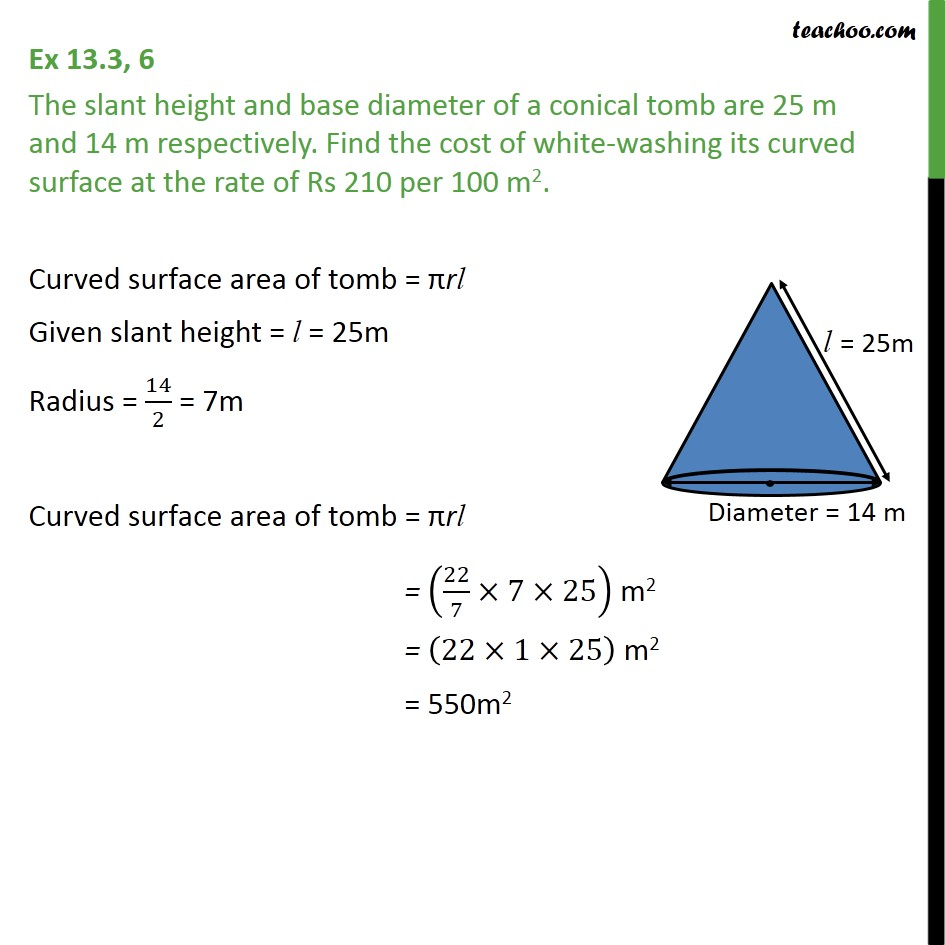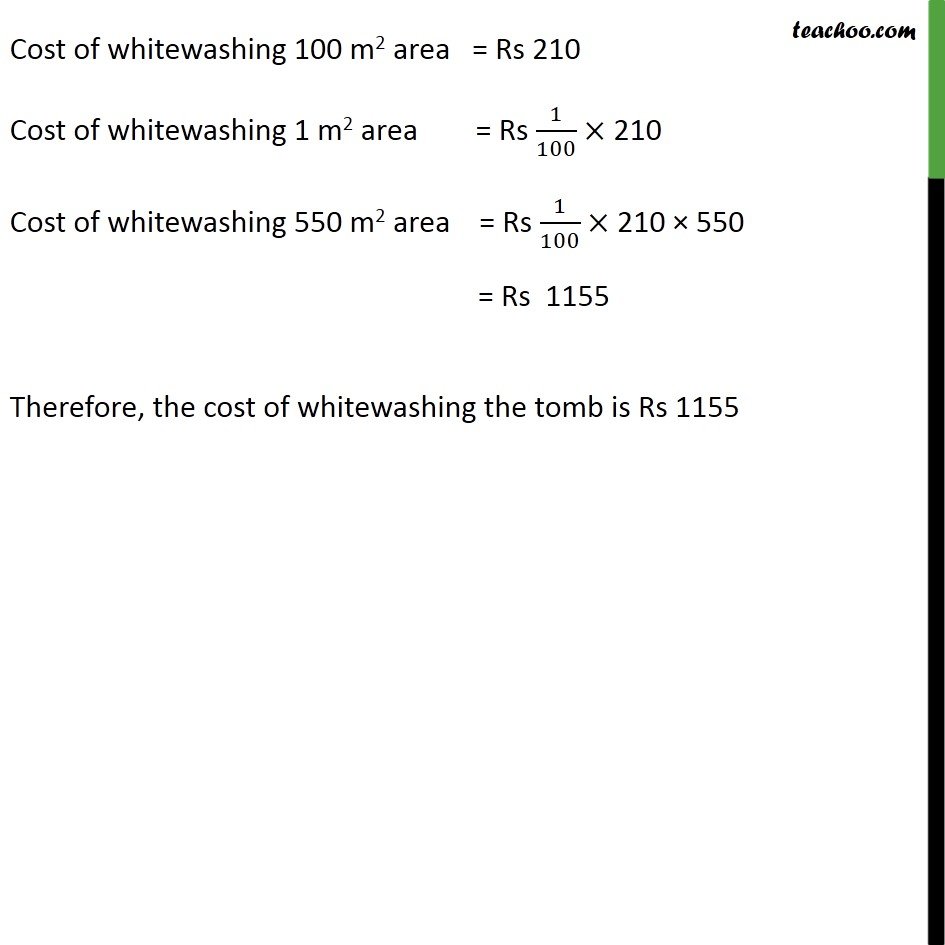Ex 11.1

Chapter 11 Class 9 Surface Areas and Volumes
Serial order wiseLearn in your speed, with individual attention - Teachoo Maths 1-on-1 Class

### Transcript

Ex 11.1, 6 The slant height and base diameter of a conical tomb are 25 m and 14 m respectively. Find the cost of white-washing its curved surface at the rate of Rs 210 per 100 m2. Curved surface area of tomb = rl Given slant height = l = 25m Radius = 14/2 = 7m Curved surface area of tomb = rl = (22/7 7 25) m2 = (22 1 25) m2 = 550m2 Cost of whitewashing 100 m2 area = Rs 210 Cost of whitewashing 1 m2 area = Rs 1/100 210 Cost of whitewashing 550 m2 area = Rs 1/100 210 550 = Rs 1155 Therefore, the cost of whitewashing the tomb is Rs 1155